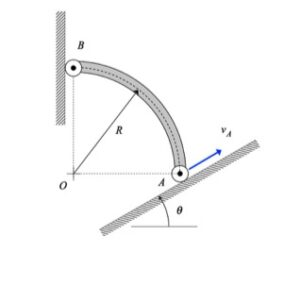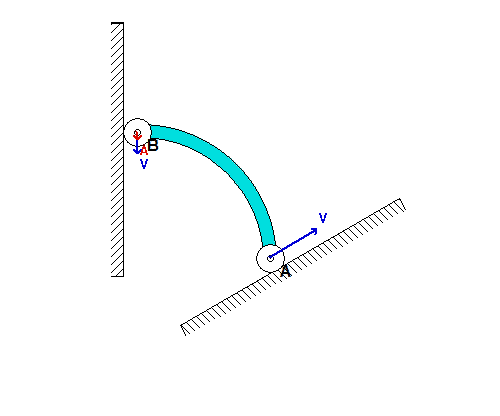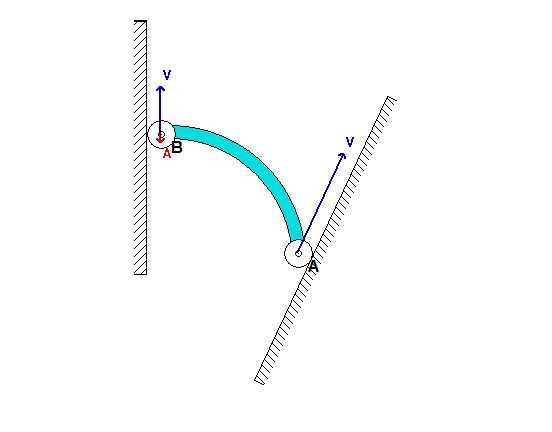# Homework H.2.CDiscussion and hints:

The solution for the velocity and acceleration of end B is a straight-forward application of the rigid body velocity and acceleration equations for member AB:

vB = vA + omega x rB/A
aB = aA + α x rB/A - ω2*rB/A

where vB = vBj_hat, aB = aB*j_hat and aA = aA*(cos(θ)*i_hat + sin(θ)*j_hat)Each of the two vector equations above represents two scalar equations, providing us with the necessary equations to solve for vB, ω, aB and α. All of the observations made above can be predicted by the above kinematics equations. Instant centers (later on in the course) can prove useful in providing explanations.For the inclination angle used in the above simulation, we see that point B moves DOWNWARD along the vertical wall as A moves up along the incline. As B moves onto the same horizontal plane as A, the acceleration of B becomes very large (although A continues to move with a constant speed). Can you provide a physical explanation for this?If we now consider a steeper inclination angle for A, as used above, we see that end B initially moves UPWARD along the wall; however, at some point B reverses its direction and begins to move DOWNWARD along the wall. Can you provide a physical explanation for this? Note also that the acceleration of B becomes very large as B moves onto the same horizontal plane as A, as it was for the initial value of inclination angle.

What is the value of the incline angle theta that defines the boundary between the types of initial motions for bar AB shown in the above two simulations? For the numerical value of the angle theta provided in the problem statement, which of the two simulations above agree with your results?

## 11 thoughts on “Homework H.2.C”

1.jone1871 says:

Wouldn't v_B and a_B be in the negative j direction?

2.Edward James Bregi says:

Not necessarily. The models shown above do a pretty solid job of describing the possible outcomes. It really depends on how steep the incline is

3.ecasetti says:

What is the acceleration of A? They aren't shown on the models, but I'm not sure that we can assume it is 0.

1.CMK says:

Escetti: Please note that the problem statement says that A moves with a constant speed. Because of this, and the fact that A moves on a straight path, the acceleration of A is zero throughout.

4.gopalakh says:

What would rB/A be for solving for the V_b?

1.CMK says:

Gopalakh: The vector r_B/A is the vector that extends from point A to point B. For the position shown, you need to move a distance of R in the -x direction and a distance of R in the +y direction in order to go from A to B.

5.qaustin says:

How do we figure out what omega is?

1.Flynn Botkin says:

If you go through finding the velocity of B in terms of A, you get an answer that has both i and j components. In order to find omega, set the i component to 0, because B is constrained to move vertically.

6.Ethan Chu says:

I don't quite see how we can find the exact values of Vb and Ab without values of Va and Aa. Are we to assume the only thing affecting the arc is gravity?

1.Ethan Chu says:

Wait sry, I know Aa is constant but still don;t know what to do with Va.

1.Cullen Barber says:

You know that A moves along an incline of angle theta, so you are able to figure out the x and y components of A's velocity in terms of Va. From there you can analyze the Vb = Va + W x Rb/a equation in the i_hat and j_hat directions, because you know that Vb is in the - j_hat direction.